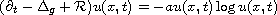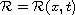Electron. J. Diff. Equ., Vol. 2015 (2015), No. 12, pp. 1-11.

### Gradient estimates for a nonlinear parabolic equation with potential under geometric flow Abimbola Abolarinwa

Abstract:
Let (M,g) be an n dimensional complete Riemannian manifold. In this article we prove local Li-Yau type gradient estimates for all positive solutions to the nonlinear parabolic equationalong the generalised geometric flow on M. Hereis a smooth potential function and a is an arbitrary constant. As an application we derive a global estimate and a space-time Harnack inequality.

Submitted December 8, 2014. Published January 8, 2015.
Math Subject Classifications: 35K55, 53C21, 53C44, 58J35.
Key Words: Gradient estimates; Harnack inequalities; parabolic equations; geometric flows.

Show me the PDF file (232 KB), TEX file, and other files for this article.Abimbola Abolarinwa Department of Mathematics, University of Sussex Brighton, BN1 9QH, United Kingdom email: A.Abolarinwa@sussex.ac.uk